# Exploratory Spatial Data Analysis (ESDA)

IPYNB

%matplotlib inline
import pysal as ps
import pandas as pd
import numpy as np
from pysal.contrib.viz import mapping as maps


A well-used functionality in PySAL is the use of PySAL to conduct exploratory spatial data analysis. This notebook will provide an overview of ways to conduct exploratory spatial analysis in Python.

First, let's read in some data:

data = ps.pdio.read_files("../data/texas.shp")

data.head()

NAME STATE_NAME STATE_FIPS CNTY_FIPS FIPS STFIPS COFIPS FIPSNO SOUTH HR60 ... BLK90 GI59 GI69 GI79 GI89 FH60 FH70 FH80 FH90 geometry
0 Lipscomb Texas 48 295 48295 48 295 48295 1 0.0 ... 0.031817 0.286929 0.378219 0.407005 0.373005 6.724512 4.5 3.835360 6.093580 <pysal.cg.shapes.Polygon object at 0x7fd6da8ad...
1 Sherman Texas 48 421 48421 48 421 48421 1 0.0 ... 0.139958 0.288976 0.359377 0.415453 0.378041 5.665722 1.7 3.253796 3.869407 <pysal.cg.shapes.Polygon object at 0x7fd6da8ad...
2 Dallam Texas 48 111 48111 48 111 48111 1 0.0 ... 2.050906 0.331667 0.385996 0.370037 0.376015 7.546049 7.2 9.471366 14.231738 <pysal.cg.shapes.Polygon object at 0x7fd6da8ad...
3 Hansford Texas 48 195 48195 48 195 48195 1 0.0 ... 0.000000 0.253527 0.357813 0.393938 0.383924 7.591786 4.7 5.542986 7.125457 <pysal.cg.shapes.Polygon object at 0x7fd6da8ad...
4 Ochiltree Texas 48 357 48357 48 357 48357 1 0.0 ... 0.021911 0.236998 0.352940 0.343949 0.374461 5.172414 4.0 4.758392 9.159159 <pysal.cg.shapes.Polygon object at 0x7fd6da8ad...

5 rows × 70 columns

import matplotlib.pyplot as plt

import geopandas as gpd
hr10 = ps.Quantiles(data.HR90, k=10)
f, ax = plt.subplots(1, figsize=(9, 9))
tx.assign(cl=hr10.yb).plot(column='cl', categorical=True, \
k=10, cmap='OrRd', linewidth=0.1, ax=ax, \
edgecolor='white', legend=True)
ax.set_axis_off()
plt.title("HR90 Deciles")
plt.show()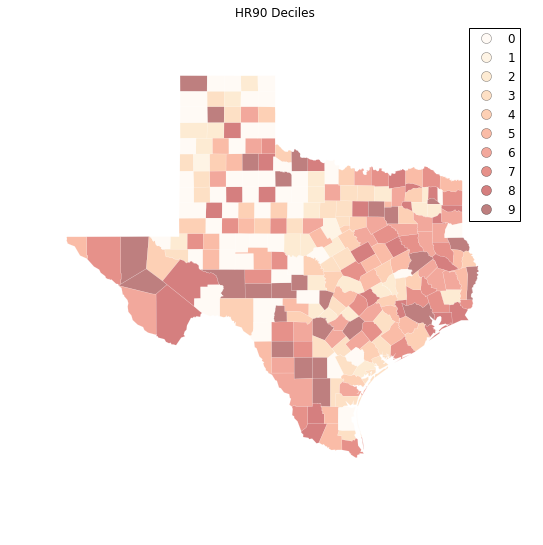## Spatial Autocorrelation

Visual inspection of the map pattern for HR90 deciles allows us to search for spatial structure. If the spatial distribution of the rates was random, then we should not see any clustering of similar values on the map. However, our visual system is drawn to the darker clusters in the south west as well as the east, and a concentration of the lighter hues (lower homicide rates) moving north to the pan handle.

Our brains are very powerful pattern recognition machines. However, sometimes they can be too powerful and lead us to detect false positives, or patterns where there are no statistical patterns. This is a particular concern when dealing with visualization of irregular polygons of differning sizes and shapes.

The concept of spatial autocorrelation relates to the combination of two types of similarity: spatial similarity and attribute similarity. Although there are many different measures of spatial autocorrelation, they all combine these two types of simmilarity into a summary measure.

Let's use PySAL to generate these two types of similarity measures.

### Spatial Similarity

We have already encountered spatial weights in a previous notebook. In spatial autocorrelation analysis, the spatial weights are used to formalize the notion of spatial similarity. As we have seen there are many ways to define spatial weights, here we will use queen contiguity:


W = ps.queen_from_shapefile("../data/texas.shp")
W.transform = 'r'


### Attribute Similarity

So the spatial weight between counties $i$ and $j$ indicates if the two counties are neighbors (i.e., geographically similar). What we also need is a measure of attribute similarity to pair up with this concept of spatial similarity. The spatial lag is a derived variable that accomplishes this for us. For county $i$ the spatial lag is defined as: $HR90Lag_i = \sum_j w_{i,j} HR90_j$

HR90Lag = ps.lag_spatial(W, data.HR90)

HR90LagQ10 = ps.Quantiles(HR90Lag, k=10)

f, ax = plt.subplots(1, figsize=(9, 9))
tx.assign(cl=HR90LagQ10.yb).plot(column='cl', categorical=True, \
k=10, cmap='OrRd', linewidth=0.1, ax=ax, \
edgecolor='white', legend=True)
ax.set_axis_off()
plt.title("HR90 Spatial Lag Deciles")

plt.show()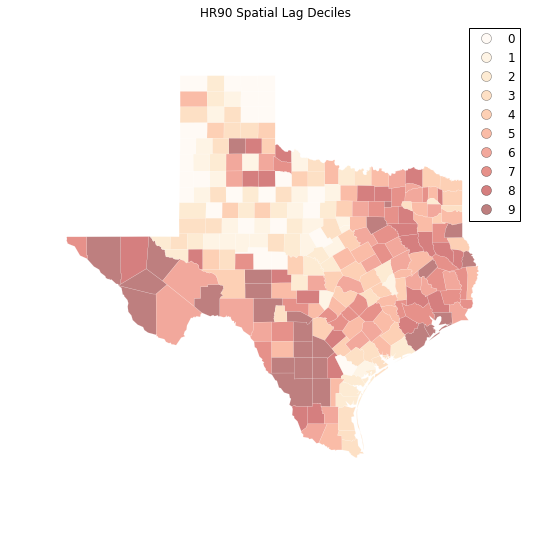The decile map for the spatial lag tends to enhance the impression of value similarity in space. However, we still have the challenge of visually associating the value of the homicide rate in a county with the value of the spatial lag of rates for the county. The latter is a weighted average of homicide rates in the focal county's neighborhood.

To complement the geovisualization of these associations we can turn to formal statistical measures of spatial autocorrelation.

HR90 = data.HR90
b,a = np.polyfit(HR90, HR90Lag, 1)

f, ax = plt.subplots(1, figsize=(9, 9))

plt.plot(HR90, HR90Lag, '.', color='firebrick')

# dashed vert at mean of the last year's PCI
plt.vlines(HR90.mean(), HR90Lag.min(), HR90Lag.max(), linestyle='--')
# dashed horizontal at mean of lagged PCI
plt.hlines(HR90Lag.mean(), HR90.min(), HR90.max(), linestyle='--')

# red line of best fit using global I as slope
plt.plot(HR90, a + b*HR90, 'r')
plt.title('Moran Scatterplot')
plt.ylabel('Spatial Lag of HR90')
plt.xlabel('HR90')
plt.show()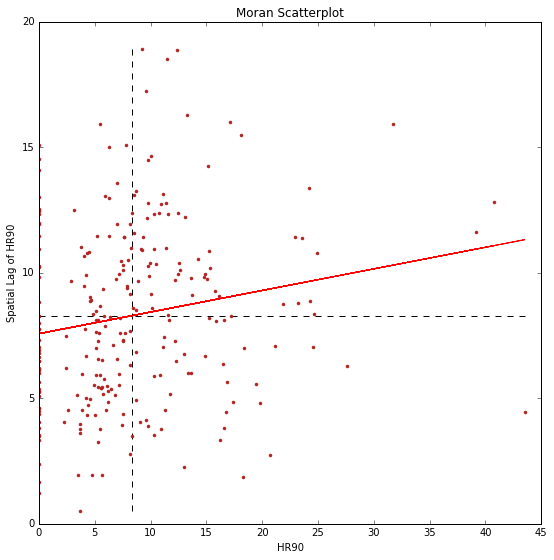## Global Spatial Autocorrelation

In PySAL, commonly-used analysis methods are very easy to access. For example, if we were interested in examining the spatial dependence in HR90 we could quickly compute a Moran's $I$ statistic:

I_HR90 = ps.Moran(data.HR90.values, W)

I_HR90.I, I_HR90.p_sim

(0.085976640313889768, 0.012999999999999999)


Thus, the $I$ statistic is $0.859$ for this data, and has a very small $p$ value.

b # note I is same as the slope of the line in the scatterplot

0.085976640313889505


We can visualize the distribution of simulated $I$ statistics using the stored collection of simulated statistics:

I_HR90.sim[0:5]

array([-0.05640543, -0.03158917,  0.0277026 ,  0.03998822, -0.01140814])


A simple way to visualize this distribution is to make a KDEplot (like we've done before), and add a rug showing all of the simulated points, and a vertical line denoting the observed value of the statistic:

import matplotlib.pyplot as plt
import seaborn as sns
%matplotlib inline

sns.kdeplot(I_HR90.sim, shade=True)
plt.vlines(I_HR90.sim, 0, 0.5)
plt.vlines(I_HR90.I, 0, 10, 'r')
plt.xlim([-0.15, 0.15])

/home/serge/anaconda2/envs/gds-scipy16/lib/python3.5/site-packages/statsmodels/nonparametric/kdetools.py:20: VisibleDeprecationWarning: using a non-integer number instead of an integer will result in an error in the future
y = X[:m/2+1] + np.r_[0,X[m/2+1:],0]*1j

(-0.15, 0.15)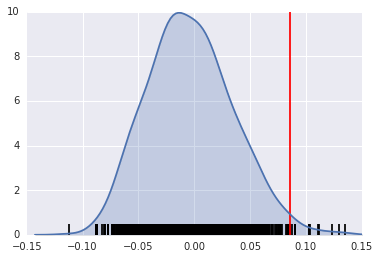Instead, if our $I$ statistic were close to our expected value, I_HR90.EI, our plot might look like this:

sns.kdeplot(I_HR90.sim, shade=True)
plt.vlines(I_HR90.sim, 0, 1)
plt.vlines(I_HR90.EI+.01, 0, 10, 'r')
plt.xlim([-0.15, 0.15])

/home/serge/anaconda2/envs/gds-scipy16/lib/python3.5/site-packages/statsmodels/nonparametric/kdetools.py:20: VisibleDeprecationWarning: using a non-integer number instead of an integer will result in an error in the future
y = X[:m/2+1] + np.r_[0,X[m/2+1:],0]*1j

(-0.15, 0.15)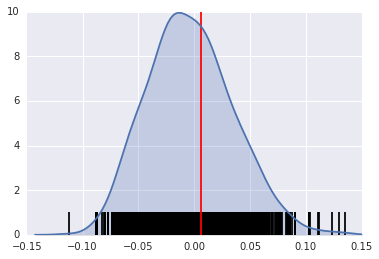The result of applying Moran's I is that we conclude the map pattern is not spatially random, but instead there is a signficant spatial association in homicide rates in Texas counties in 1990.

This result applies to the map as a whole, and is sometimes referred to as "global spatial autocorrelation". Next we turn to a local analysis where the attention shifts to detection of hot spots, cold spots and spatial outliers.

## Local Autocorrelation Statistics

In addition to the Global autocorrelation statistics, PySAL has many local autocorrelation statistics. Let's compute a local Moran statistic for the same data shown above:

LMo_HR90 = ps.Moran_Local(data.HR90.values, W)


Now, instead of a single $I$ statistic, we have an array of local $I_i$ statistics, stored in the .Is attribute, and p-values from the simulation are in p_sim.

LMo_HR90.Is[0:10], LMo_HR90.p_sim[0:10]

(array([ 1.12087323,  0.47485223, -1.22758423,  0.93868661,  0.68974296,
0.78503173,  0.71047515,  0.41060686,  0.00740368,  0.14866352]),
array([ 0.013,  0.169,  0.037,  0.015,  0.002,  0.009,  0.053,  0.063,
0.489,  0.119]))


We can adjust the number of permutations used to derive every pseudo-$p$ value by passing a different permutations argument:

LMo_HR90 = ps.Moran_Local(data.HR90.values, W, permutations=9999)


In addition to the typical clustermap, a helpful visualization for LISA statistics is a Moran scatterplot with statistically significant LISA values highlighted.

This is very simple, if we use the same strategy we used before:

First, construct the spatial lag of the covariate:

Lag_HR90 = ps.lag_spatial(W, data.HR90.values)
HR90 = data.HR90.values


Then, we want to plot the statistically-significant LISA values in a different color than the others. To do this, first find all of the statistically significant LISAs. Since the $p$-values are in the same order as the $I_i$ statistics, we can do this in the following way

sigs = HR90[LMo_HR90.p_sim <= .001]
W_sigs = Lag_HR90[LMo_HR90.p_sim <= .001]
insigs = HR90[LMo_HR90.p_sim > .001]
W_insigs = Lag_HR90[LMo_HR90.p_sim > .001]


Then, since we have a lot of points, we can plot the points with a statistically insignficant LISA value lighter using the alpha keyword. In addition, we would like to plot the statistically significant points in a dark red color.

b,a = np.polyfit(HR90, Lag_HR90, 1)


Matplotlib has a list of named colors and will interpret colors that are provided in hexadecimal strings:

plt.plot(sigs, W_sigs, '.', color='firebrick')
plt.plot(insigs, W_insigs, '.k', alpha=.2)
# dashed vert at mean of the last year's PCI
plt.vlines(HR90.mean(), Lag_HR90.min(), Lag_HR90.max(), linestyle='--')
# dashed horizontal at mean of lagged PCI
plt.hlines(Lag_HR90.mean(), HR90.min(), HR90.max(), linestyle='--')

# red line of best fit using global I as slope
plt.plot(HR90, a + b*HR90, 'r')
plt.text(s='$I = %.3f$' % I_HR90.I, x=50, y=15, fontsize=18)
plt.title('Moran Scatterplot')
plt.ylabel('Spatial Lag of HR90')
plt.xlabel('HR90')

<matplotlib.text.Text at 0x7fd6cf324d30>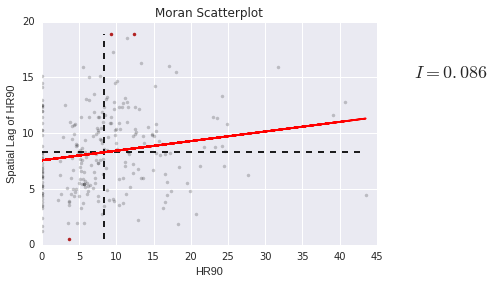We can also make a LISA map of the data.

sig = LMo_HR90.p_sim < 0.05

sig.sum()

44

hotspots = LMo_HR90.q==1 * sig

hotspots.sum()

10

coldspots = LMo_HR90.q==3 * sig

coldspots.sum()

17

data.HR90[hotspots]

98      9.784698
132    11.435106
164    17.129154
166    11.148272
209    13.274924
229    12.371338
234    31.721863
236     9.584971
239     9.256549
242    18.062652
Name: HR90, dtype: float64

data[hotspots]

NAME STATE_NAME STATE_FIPS CNTY_FIPS FIPS STFIPS COFIPS FIPSNO SOUTH HR60 ... BLK90 GI59 GI69 GI79 GI89 FH60 FH70 FH80 FH90 geometry
98 Ellis Texas 48 139 48139 48 139 48139 1 9.217652 ... 10.009746 0.325785 0.365177 0.352516 0.372783 12.418831 10.5 9.076165 12.031635 <pysal.cg.shapes.Polygon object at 0x7fd6cc81a...
132 Hudspeth Texas 48 229 48229 48 229 48229 1 9.971084 ... 0.514580 0.312484 0.373474 0.440944 0.476631 14.115899 7.7 8.959538 11.363636 <pysal.cg.shapes.Polygon object at 0x7fd6cc7a1...
164 Jeff Davis Texas 48 243 48243 48 243 48243 1 0.000000 ... 0.359712 0.316019 0.367719 0.437014 0.399655 14.438503 10.1 5.970149 8.255159 <pysal.cg.shapes.Polygon object at 0x7fd6cc7ae...
166 Schleicher Texas 48 413 48413 48 413 48413 1 0.000000 ... 0.903010 0.300170 0.387936 0.419192 0.419375 10.155148 9.8 7.222914 8.363636 <pysal.cg.shapes.Polygon object at 0x7fd6cc7ae...
209 Chambers Texas 48 071 48071 48 71 48071 1 3.211613 ... 12.694146 0.299847 0.374105 0.378431 0.364723 9.462037 9.2 8.568120 10.598911 <pysal.cg.shapes.Polygon object at 0x7fd6cc7bb...
229 Frio Texas 48 163 48163 48 163 48163 1 3.296414 ... 1.358373 0.390980 0.463020 0.435098 0.473507 14.665445 9.4 11.842919 18.330362 <pysal.cg.shapes.Polygon object at 0x7fd6cc7c2...
234 La Salle Texas 48 283 48283 48 283 48283 1 0.000000 ... 1.008755 0.421556 0.482174 0.489173 0.492687 18.167702 14.1 13.052937 20.088626 <pysal.cg.shapes.Polygon object at 0x7fd6cc7c2...
236 Dimmit Texas 48 127 48127 48 127 48127 1 0.000000 ... 0.575098 0.417976 0.452789 0.456840 0.479503 13.826043 10.1 10.944363 17.769080 <pysal.cg.shapes.Polygon object at 0x7fd6cc7c2...
239 Webb Texas 48 479 48479 48 479 48479 1 2.057899 ... 0.117083 0.382594 0.443082 0.439100 0.461075 20.292824 15.5 17.419676 20.521271 <pysal.cg.shapes.Polygon object at 0x7fd6cc7c2...
242 Duval Texas 48 131 48131 48 131 48131 1 2.487934 ... 0.092894 0.370217 0.427660 0.421041 0.458937 15.829478 13.2 12.803677 20.699881 <pysal.cg.shapes.Polygon object at 0x7fd6cc7c2...

10 rows × 70 columns

from matplotlib import colors
hmap = colors.ListedColormap(['grey', 'red'])
f, ax = plt.subplots(1, figsize=(9, 9))
tx.assign(cl=hotspots*1).plot(column='cl', categorical=True, \
k=2, cmap=hmap, linewidth=0.1, ax=ax, \
edgecolor='grey', legend=True)
ax.set_axis_off()
plt.show()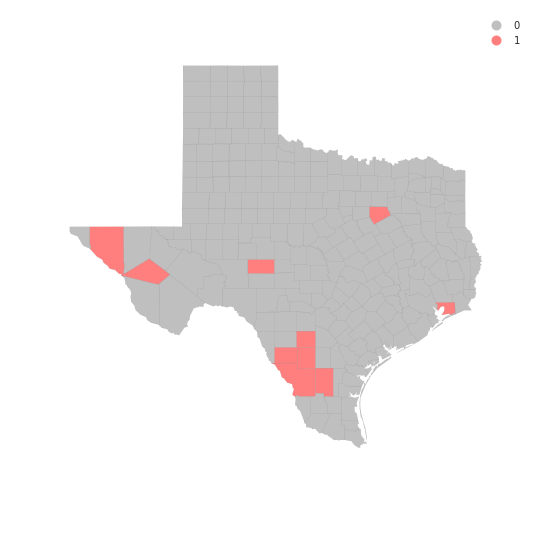data.HR90[coldspots]

0      0.000000
3      0.000000
4      3.651767
5      0.000000
13     5.669899
19     3.480743
21     3.675119
32     2.211607
33     4.718762
48     5.509870
51     0.000000
62     3.677958
69     0.000000
81     0.000000
87     3.699593
140    8.125292
233    5.304688
Name: HR90, dtype: float64

cmap = colors.ListedColormap(['grey', 'blue'])
f, ax = plt.subplots(1, figsize=(9, 9))
tx.assign(cl=coldspots*1).plot(column='cl', categorical=True, \
k=2, cmap=cmap, linewidth=0.1, ax=ax, \
edgecolor='black', legend=True)
ax.set_axis_off()
plt.show()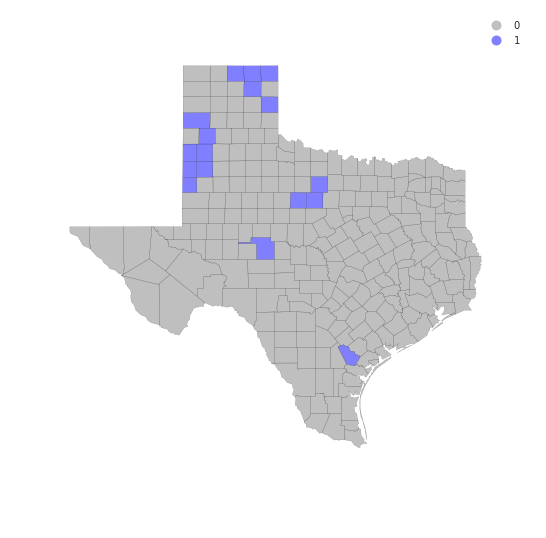from matplotlib import colors
hcmap = colors.ListedColormap(['grey', 'red','blue'])
hotcold = hotspots*1 + coldspots*2
f, ax = plt.subplots(1, figsize=(9, 9))
tx.assign(cl=hotcold).plot(column='cl', categorical=True, \
k=2, cmap=hcmap,linewidth=0.1, ax=ax, \
edgecolor='black', legend=True)
ax.set_axis_off()
plt.show()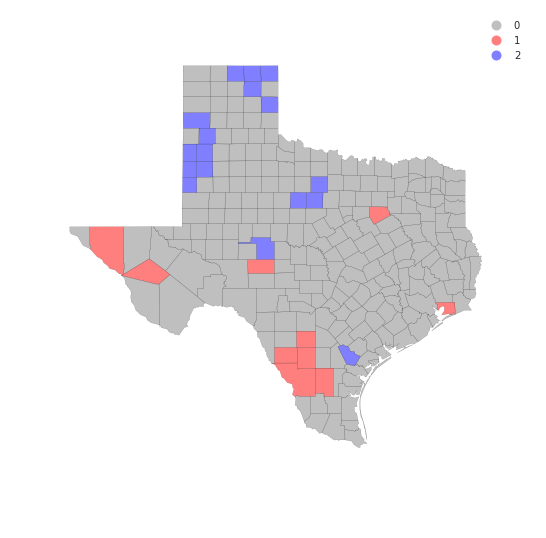sns.kdeplot(data.HR90)

/home/serge/anaconda2/envs/gds-scipy16/lib/python3.5/site-packages/statsmodels/nonparametric/kdetools.py:20: VisibleDeprecationWarning: using a non-integer number instead of an integer will result in an error in the future
y = X[:m/2+1] + np.r_[0,X[m/2+1:],0]*1j

<matplotlib.axes._subplots.AxesSubplot at 0x7fd6ccc17358>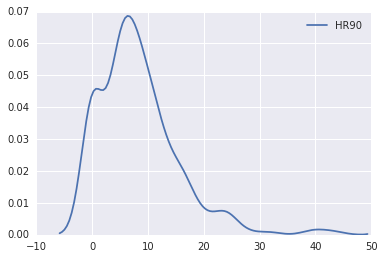sns.kdeplot(data.HR90)
sns.kdeplot(data.HR80)
sns.kdeplot(data.HR70)
sns.kdeplot(data.HR60)

/home/serge/anaconda2/envs/gds-scipy16/lib/python3.5/site-packages/statsmodels/nonparametric/kdetools.py:20: VisibleDeprecationWarning: using a non-integer number instead of an integer will result in an error in the future
y = X[:m/2+1] + np.r_[0,X[m/2+1:],0]*1j

<matplotlib.axes._subplots.AxesSubplot at 0x7fd6da838908>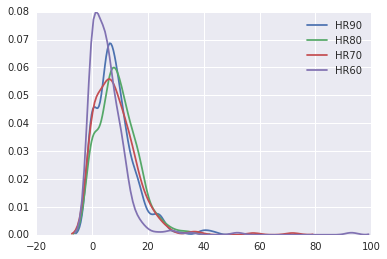data.HR90.mean()

8.302494460285041

data.HR90.median()

7.23234613355


## Exercises

1. Repeat the global analysis for the years 1960, 70, 80 and compare the results to what we found in 1990.
2. The local analysis can also be repeated for the other decades. How many counties are hot spots in each of the periods?
3. The recent Brexit vote provides a timely example where local spatial autocorrelation analysis can provide interesting insights. One local analysis of the vote to leave has recently been repored. Extend this to do an analysis of the attribute Pct_remain. Do the hot spots for the leave vote concord with the cold spots for the remain vote?